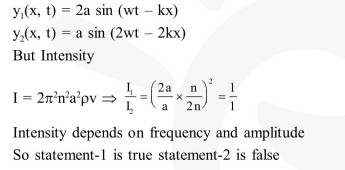Deepak Scored 45->99%ile with Bounce Back Crack Course. You can do it too!

# Solve this following

Question:

Statement-1: Two longitudinal waves given by equations : $\mathrm{y}_{1}(\mathrm{x}, \mathrm{t})=2 \mathrm{a} \sin (\omega \mathrm{t}-\mathrm{kx})$ and $\mathrm{y}_{2}(\mathrm{x}, \mathrm{t})=$ a $\sin (2 \omega t-2 k x)$ will have equal intensity.

Statement-1: Intensity of waves of given frequency in same medium is proportional to square of amplitude only.

1. Statement- 1 is false, statement- 2 is true

2. Statement-1 is ture, statement- 2 is false

3. Statement- 1 is ture, statement- 2 true; statement- 2 is the correct explanation of statement- 1

4. Statement- 1 is true, statement- 2 is true; statement $-2$ is not correct explanation of statement- 1 .

Correct Option: , 2

Solution: